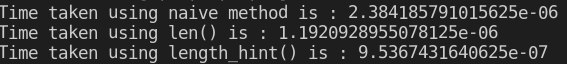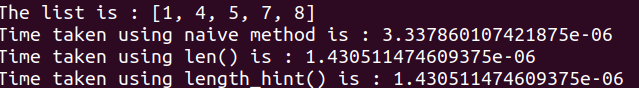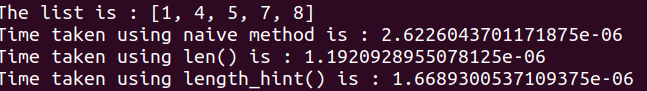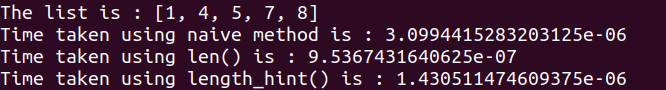Open In App
Related Articles
• Write an Interview Experience
• Python Programs

# How To Find the Length of a List in Python

List being an integral part of Python day-to-day programming has to be learned by all Python users and having a knowledge of its utility and operations is essential and always a plus. So this article discusses one such utility of finding the no. of elements in a list using Python.

Example:

`Input: lst = [10,20,30,40]Output: 4Explanation: The output is 4 because the length of the list is  4.`

## Find the Length of a List in Python

### Get the Length of a List in Python using len()

Python len() function is an inbuilt function in Python. It can be used to find the length of an object by passing the object within the parentheses of the len function.

## Python3

 `# Python len()``li ``=` `[``10``, ``20``, ``30``]``n ``=` `len``(li)``print``(``"The length of list is: "``, n)`

Output:

`The length of list is: 3`

Time Complexity: O(n), where n is the length of the list
Auxiliary Space: O(1)

### Find the Length of a List in Python Naive Method

In this method, one just runs a loop and increases the counter till the last element of the list to know its count. This is the most basic strategy that can be possibly employed in the absence of other present techniques.

## Python3

 `# Python code to demonstrate``# length of list``# using naive method` `# Initializing list``test_list ``=` `[``1``, ``4``, ``5``, ``7``, ``8``]` `# Printing test_list``print``(``"The list is : "` `+` `str``(test_list))` `# Finding length of list``# using loop``# Initializing counter``counter ``=` `0``for` `i ``in` `test_list:` `    ``# incrementing counter``    ``counter ``=` `counter ``+` `1` `# Printing length of list``print``(``"Length of list using naive method is : "` `+` `str``(counter))`

Output:

`The list is : [1, 4, 5, 7, 8]Length of list using naive method is : 5`

Time Complexity: O(n)
Auxiliary Space: O(1)

### Find the Length of a List in Python using length_hint()

This technique is a lesser-known technique for finding list length. This particular method is defined in the operator class and it can also tell the no. of elements present in the list. Here, we are finding length of list using len() and length_hint()

## Python3

 `# Python code to demonstrate``# length of list``# using len() and length_hint``from` `operator ``import` `length_hint` `# Initializing list``test_list ``=` `[``1``, ``4``, ``5``, ``7``, ``8``]` `# Printing test_list``print``(``"The list is : "` `+` `str``(test_list))` `# Finding length of list``# using len()``list_len ``=` `len``(test_list)` `# Finding length of list``# using length_hint()``list_len_hint ``=` `length_hint(test_list)` `# Printing length of list``print``(``"Length of list using len() is : "` `+` `str``(list_len))``print``(``"Length of list using length_hint() is : "` `+` `str``(list_len_hint))`

Output :

`The list is : [1, 4, 5, 7, 8]Length of list using len() is : 5Length of list using length_hint() is : 5`

### Find the Length of a List in Python using sum()

Use iteration inside the sum and with each iteration adds one and at the end of the iteration, we get the total length of the list.

## Python3

 `# Python code to demonstrate``# length of list``# using sum()` `# Initializing list``test_list ``=` `[``1``, ``4``, ``5``, ``7``, ``8``]` `# Printing test_list``print``(``"The list is : "` `+` `str``(test_list))` `# Finding length of list``# using sum()``list_len ``=` `sum``(``1` `for` `i ``in` `test_list)`  `# Printing length of list``print``(``"Length of list using len() is : "` `+` `str``(list_len))``print``(``"Length of list using length_hint() is : "` `+` `str``(list_len))`

Output:

`The list is : [1, 4, 5, 7, 8]Length of list using len() is : 5Length of list using length_hint() is : 5`

### Find the Length of a List in Python using a List Comprehension

Initialize a list called test_list with some values then Initialize a variable called length to 0. Use a list comprehension to generate a sequence of ones for each element in the test_list. This will create a list of ones with the same length as the test_list. Now use the sum() function to sum all the ones in the list generated by the list comprehension. Assign the sum to the length variable. Print the length variable.

## Python3

 `# Define the list to be used for the demonstration``test_list ``=` `[``1``, ``4``, ``5``, ``7``, ``8``]` `# Calculate the length of the list using a list comprehension and the sum function``# The list comprehension generates a sequence of ones for each element in the list``# The sum function then sums all the ones to give the length of the list``length ``=` `sum``(``1` `for` `_ ``in` `test_list)` `# Print the length of the list``print``(``"Length of list using list comprehension is:"``, length)`

Output

```Length of list using list comprehension is: 5

```

Time Complexity: The list comprehension creates a new list with a length equal to the length of the test_list. The sum() function then iterates over this list to compute the sum. Therefore, the time complexity of this algorithm is O(N), where N is the length of the test_list.

Auxiliary Space: The algorithm creates a new list of ones with a length equal to the length of the test_list using the list comprehension. Therefore, the auxiliary space complexity is also O(N), where N is the length of the test_list.

### Find the Length of a List in Python using recursion

This function takes a list lst as input and recursively calls itself, passing in a slice of the list that excludes the first element until the list is empty. The base case is when the list is empty, in which case the function returns 0. Otherwise, it adds 1 to the result of calling the function on the rest of the list.

## Python3

 `# Define a function to count the number of elements in a list using recursion``def` `count_elements_recursion(lst):``    ``# Base case: if the list is empty, return 0``    ``if` `not` `lst:``        ``return` `0``    ``# Recursive case: add 1 to the count of the remaining elements in the list``    ``return` `1` `+` `count_elements_recursion(lst[``1``:])`  `# Test the function with a sample list``lst ``=` `[``1``, ``2``, ``3``, ``4``, ``5``]``print``(``"The length of the list is:"``, count_elements_recursion(lst))` `# Output: The length of the list is: 5`

Output

```The length of the list is: 5

```

Time complexity: O(n) where n is the length of the list. This is because the function makes n recursive calls, each taking O(1) time, and there is also O(1) work done at each level outside of the recursive call.

Space complexity: O(n) where n is the length of the list. This is because the function creates n stack frames on the call stack due to the recursive calls.

### Find the Length of a List in Python using enumerate function

Python Enumerate() method adds a counter to an iterable and returns it in a form of an enumerating object.

## Python3

 `# python code to find the length``# of list using enumerate function``list1 ``=` `[``1``, ``4``, ``5``, ``7``, ``8``]``s ``=` `0``for` `i, a ``in` `enumerate``(list1):``    ``s ``+``=` `1``print``(s)`

Output

```5

```

### Find the Length of a List in Python using Collections

Alternatively, you can also use the sum() function along with the values() method of the Collections Counter object to get the length of the list.

## Python3

 `from` `collections ``import` `Counter` `# Initializing list``test_list ``=` `[``1``, ``4``, ``5``, ``7``, ``8``]` `# Finding length of list using Counter()``list_len ``=` `sum``(Counter(test_list).values())` `print``(``"Length of list using Counter() is:"``, list_len)``# This code is contributed by Edula Vinay Kumar Reddy`

Output

```Length of list using Counter() is: 5

```

Time complexity: O(n), where n is the length of the list. This is because the Counter() function has a time complexity of O(n) when applied to a list of length n, and the values() method and the sum() function both have a time complexity of O(n) when applied to a list of length n.

The space complexity: O(n), as the Counter() function, creates a dictionary with n key-value pairs, each representing an element and its count in the list, respectively. This dictionary takes up O(n) space.

## Performance Analysis

### Naive vs Python len() vs Python length_hint()

When choosing amongst alternatives it’s always necessary to have a valid reason why to choose one over another. This section does a time analysis of how much time it takes to execute all of them to offer a better choice to use.

## Python3

 `from` `operator ``import` `length_hint``import` `time` `# Initializing list``test_list ``=` `[``1``, ``4``, ``5``, ``7``, ``8``]` `# Printing test_list``print``(``"The list is : "` `+` `str``(test_list))` `# Finding length of list``# using loop``# Initializing counter``start_time_naive ``=` `time.time()``counter ``=` `0``for` `i ``in` `test_list:` `    ``# incrementing counter``    ``counter ``=` `counter ``+` `1``end_time_naive ``=` `str``(time.time() ``-` `start_time_naive)` `# Finding length of list``# using len()``start_time_len ``=` `time.time()``list_len ``=` `len``(test_list)``end_time_len ``=` `str``(time.time() ``-` `start_time_len)` `# Finding length of list``# using length_hint()``start_time_hint ``=` `time.time()``list_len_hint ``=` `length_hint(test_list)``end_time_hint ``=` `str``(time.time() ``-` `start_time_hint)` `# Printing Times of each``print``(``"Time taken using naive method is : "` `+` `end_time_naive)``print``(``"Time taken using len() is : "` `+` `end_time_len)``print``(``"Time taken using length_hint() is : "` `+` `end_time_hint)`

Output:

`The list is : [1, 4, 5, 7, 8]Time taken using naive method is : 2.6226043701171875e-06Time taken using len() is : 1.1920928955078125e-06Time taken using length_hint() is : 1.430511474609375e-06`

In the below images, it can be clearly seen that time taken is naive >> length_hint() > len(), but the time taken depends highly on the OS and several of its parameter. In two consecutive runs, you may get contrasting results, in fact sometimes naive takes the least time out of three. All the possible 6 permutations are possible.naive > len() > length_hint()naive > len()=length_hint()naive > length_hint() >len()naive > length_hint()  > len()